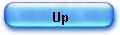The Difference Method of Henry Briggs

Briggs presented in Arithmetica logarithmica the different methods he used to compute decimal logarithms and build his tables, Briggs considered several methods, each one improving computing efficiency and reducing calculation time :

CH 6 'Continued means' (repeated square roots) method

CH 8 : Difference method

CH 11 Proportional parts (interpolation) in the linear region

CH 12 Sub tabulation (1st method) using second differences,

CH 13 Sub tabulation (2nd method - finite difference method)

Chapter 6 : the main method is covered in the page 'Briggs and the HP35'.

The 2 following texts are about 'Difference methods' (Chap 8, 12 & 13).

Chapter 14 (Radix method), Chapter 11 and Oughtred's Appendix are convered in 'The Radix Method'.

\$1 - The Henry Briggs’ Difference Method Story,
by Erik Vestergaard.

The story about Henry Briggs’ Difference Method, as described in Chapter 13 of Arithmetica Logaritmica, London 1624, is the story about a brilliant method invented to help calculate a table of logarithms. But it is also a story about affront of professional pride.

In the following I will present a short description of the situation at the time of Briggs and outline the most important aspects of his methods used to calculate his logarithms.

Else I will concentrate about the difference method mentioned above and deal with in a more modern way.

In 1614 the scotch mathematician and theologian John Napier (1550–1617) had published his table of Logarithms – an important milestone in the history of mathematics. This table was immediately met with big interest, in particular by the British mathematician Henry Briggs (1561–1630), who was a professor at Gresham College, London. Briggs was very interested in applications in navigation, a field of major importance for England.

Remember the fact that Sir Francis Drake in 1588 had defeated the Spanish Armada in The British Channel and thereby prevented an invasion by the Catholic Spaniards. The power of the Navy was in other words very important in those days. One can feel Briggs’ enthusiasm for the newly invented logarithms from his letter to ArchBishop Usher, dated Gresham House, 10th of March 1615:

Neper, Lord of Markinston, hath set my head and hands a work with his new and admirable logarithms. I hope to see him this summer, if it pleases God, for I never saw book which pleased me better, and made me more wonder.

The reason why Napier’s name is spelled in a special way here is the fact that orthographic rules did not exist at that time in England. Briggs succeeded in planning a meeting with Napier and it took place in 1615. During his visit the two men discussed the possibility of redefining “Napier’s logarithm”, and they agreed that it would be convenient if the logarithm did satisfy the identities log(1) = 0 and log(1010 ) = 1010, which would result in a logarithm, which is 109 times our present logarithm of base 10. Later Briggs changed the logarithm to the one we use today! Napier, however, was sick and too weak to produce a new table, so it was Briggs fate to do so.

In 1624 Henry Briggs published the book Arithmetica Logarithmica. Besides a table consisting of the logarithms from 1 to 20000 and from 90000 to 100000, accurate to 14 decimal places, the book also contained explanations and examples on how the table had been computed – but without proofs for the validity of his methods. Briggs methods for computing logarithms are fundamentally different from those of Napier. Briggs used a whole range of different tricks. It will go too far to describe these in details here.

Besides Briggs’ own work in Latin, Arithmetica Logarithmica (), the reader is referred to [4, p. 61–75] and [3, p. 13–20, 23–32]. The outlines of Briggs’ methods should be presented here, though.

One of the pillars in the strategy of Briggs is the following discoveries:

a) The logarithm of the square root of a number t is equal to 1/2 times the logarithm of that number.
b) For x a small number log(1 + x) ~ k x  , where k is a constant. He calculated the following value for k: 0,43429448190325180...

Notice that in modern terminology b) can be expressed in the following way: The 1. degree polynomial approximating to the logarithmic function in the point xo = 1 is a good approximation to the logarithm in the neighbourhood of that point.

Briggs’ idea was to bring that number t, to which he wanted to calculate the logarithm, down to a number close to 1 by taking repeated square roots. The logarithm of the resulting number he could then find by applying b) above. To get back to log(t) he needed to multiply by 2n , where n is the number of times he extracted square roots. He used this method to calculate log(2) .

Here he applied the square root 54 times! This required of course an enormous amount of calculations. Fortunately he had invented a special difference method to reduce the amount of calculations needed. Briggs describes this procedure in chapter 8. Eventually also see [3, p. 16] or [6, p. 73].

Using this difference method and other ingenious tricks he was able to calculate the logarithm of a number of primes. Knowing the logarithms of these primes he could rather easily calculate the logarithms to a large number of composite numbers, using the product rule for logarithms.

In chapter 12 and 13 Briggs deals with interpolation methods, that is, methods to calculate function values of x-values, which are distributed evenly between values with known function values. What he does in chapter 12 actually corresponds to the method now called Newton-forward. However his real great contribution is the unusual method described in chapter 13, which show his deep understanding of Numerical Analysis.

Without actually applying it, Briggs describes how his difference method can be used to calculate the rest of his table of logarithms between 20000 and 90000: Notice that 20000 = 5 . 4000,  20005 = 5 . 4001,  20010 = 5 . 4002, ... , 90000 = 5 . 18000. Because he had already calculated the logarithms of the first 20000 natural numbers, the logarithms of could easily be calculated using the product rule. Finally he explained how the logarithms of the remaining intermediate natural numbers could be calculated using his special difference method.

20000, 20005, 20010, 20015, 20020, , 90000 …

As mentioned earlier, Briggs actually never finished his table. The Dutchman Adrain Vlacq (1600–1666 (maybe 1667)) finished the table and it was published in 1628 – see . The accuracy in Vlacq’s table was however “only” 10 decimals, against Briggs’ 14 decimals!

Briggs explanations on how the table was calculated was almost entirely repeated in Vlacq’s book, but the chapters 12 and 13 were missing!! Briggs did take this fact as an insult, as one can feel in Briggs comments, which can be read in a later work of Briggs: Trigonometria Brittanica from 1633:

Modus correctionis a me traditus est Arithmeticae Logarithmicae Capite 13, in Editione Londinensi: Istud autem Caput una cum sequenti in Editione Batava me inconsulto & inscio omissum fuit: nec in omnibus, Editionis illius Author Vir alioqui industrius & non indoctus meam mentem videtur assequutus: Ideoque ne quicquam desit cuiquam, qui integrum Canonem conficere cupiat, quaedam maximé necessaria illinc huc transferanda cencui.

Let me translate to my best:

I have described the entire correction method in chapter 13 of the Londonedition of Arithmetica Logarithmica, but this chapter and the following are omitted in the Dutch edition, without my consent and knowledge. The Author of this edition seem not to have understood my intention to the full extent, although this person is hard-working and not without wisdom. To those, who don’t want to miss something, I have found it appropriate to transfer some most necessary details from the London-edition to this edition.

Briggs did a mistake here: It is not as he mention chapter 13 and 14, which are omitted, but 12 and 13.

\$2) Detailing the ingenious "difference method",
by Jacques Laporte.

Briggs searched a way to cut down the tiresome labour in his calculations by reducing the number of direct square roots.

“Atque ad hunc modum, cuiuscunque numeri propositi Logarithmum, per continue Medios invenire poterimus. Quos nobis lateris quadrati inventio suppeditat satis laboriose. huius autem tanti laboris molestia minuetur plurimum per Differentias.

We can find the logarithm of any proposed number by continued means according to this method which supplies our need laboriously enough by finding the square root: but the vexation of this enormous labour is most lessened through differences
.”

He found his ingenious “difference method” presented in the chapter VIII of his book, “after much frustration with the long and tedious square root method of completing the square, presumably during or after the work involved in making up the successive means table, to which he alluded in the preface to the introduction to his tables.” Ian Bruce (University of Adelaide, Australia)

I will introduce the method with modern notation and taking the example of log(3).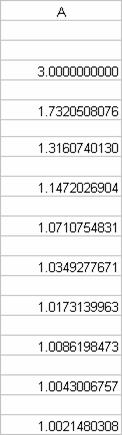Let’s suppose we have taken 7 repeated square roots up to the result 1.008619847 and we are looking to expand down the table for a better accuracy.
As a matter of fact, Briggs carried his calculation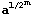to 30 decimal places, a precision that requires 50 repeated square roots: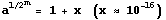.

(x is a decimal number with 15 zeros followed by 15 significant digits).

Here is the detail of the difference method.

Briggs noticed -in the repeated square root (col A) - that's the fractional parts of the numbers are roughly halved as he scrolled down the column.

Then he had the genial idea of expanding the differences to the right, by subtracting the fraction parts, e. g. line 4: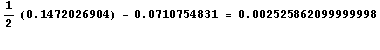forming thus the column B.

Briggs remarked that the numbers of this column B are decreasing roughly by a factor of a quarter and he continued to the right and discovered –for the other columns -that the decreasing factors were: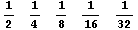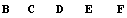Briggs continued his table until the differences in the last column diminished to zero.

Then he computed the missing numbers from right to left and filled the rest of the table, using the same procedure (in red).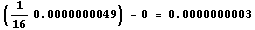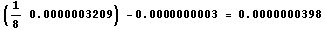,

up to the first entry in the first table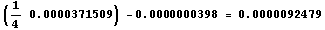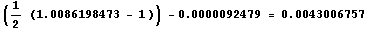=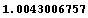in column A.

This procedure can be repeated one line at a time reducing drastically the number of calculations.
One line further we get (10th square root) =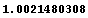in column A and x =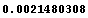,

The final result is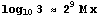(Golden rule)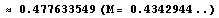Briggs’ approach is very effective practically as its convergence is fast (around 5 digits per line).

On the theoretical side, it has an enormous interest (1). As a matter of fact, the difference method consists in calculating in column A: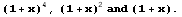and, working from left to right and right to left, line by line, to compute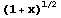.

Henry Briggs in 1624 -with a very powerful insight- finds the binomial series for a non integer number.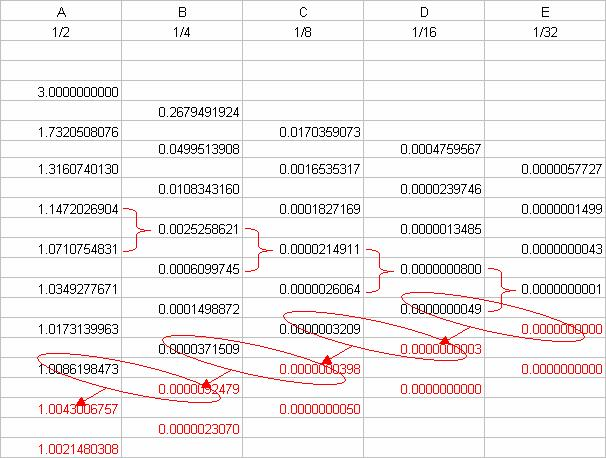For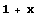itself and the lines above and below, we have:

 A B C D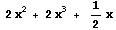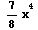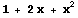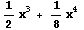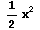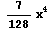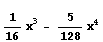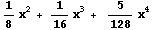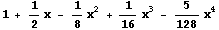Henry Briggs, Arithmetica Logarithmica. London 1624.
 Henry Briggs, Trigonometria Brittanica. Goudae, 1633.

 Ian Bruce, Arithmetica Logarithmica, Translation and annotations, Chapter 8.
 Florian Cajori, A History of Mathematics, 1893,

 Herman H. Goldstine, A History of Numerical Analysis from the 16th through the 19th Century. Springerverlag, 1977.
 Charles Hutton, Mathematical Tables (on line in Google book), 7th. edition, London, 1811.